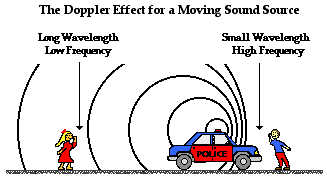# A commuter train passes a passenger platform at a constant speed of 40.0 m/s. The train horn is sounded at its frequency of 320 Hz. What wavelength is detected by a person on the platform as the train approaches?

Dec 7, 2015

${\lambda}_{o b s} = 0.949 \text{m}$

#### Explanation:

I'll assume 20 degC for which the speed of sound is $343.7 \text{m/s}$

This is an example of the Doppler Effect.

For the person standing on the platform, more wavefronts are arriving per second so he or she will detect sound of a shorter wavelength and higher frequency.If the source is approaching the observed wavelength is given by:

${\lambda}_{o b s} = \frac{\left(v - {v}_{s}\right)}{f} _ \left(\text{source}\right)$

Putting in the numbers:

${\lambda}_{o b s} = \frac{\left(343.7 - 40\right)}{320} = 0.949 \text{m}$

You can compare this to the note which someone would hear on the train:

$\lambda = \frac{v}{f} = \frac{343.7}{320} = 1.07 \text{m}$

So you can see that the person on the platform detects a slightly shorter wavelength and a slightly higher frequency of note.